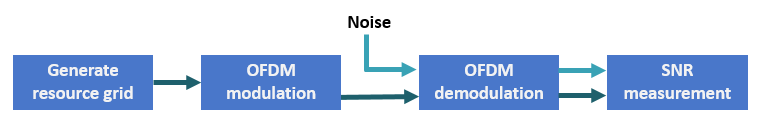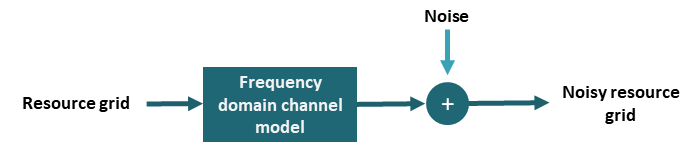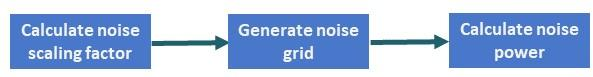# SNR Definition Used in Link Simulations

This example shows how 5G Toolbox™ link-level simulations define the signal-to-noise ratio (SNR) for time-domain and frequency-domain channel modeling.

### SNR Definition

5G Toolbox™ examples that simulate link-level simulations define the SNR as the average SNR per resource element (RE) per receive antenna. They define the REs in the resource grid, that is, in the frequency domain. To achieve the desired SNR, the examples scale the noise. Some examples use time-domain channel models, while others use frequency-domain channel models. These examples scale the noise differently depending on the channel modeling.

To define the SNR, the examples calculate it as

$\mathit{SNR}=\frac{{\mathit{S}}_{\mathrm{RE}}}{{\mathit{N}}_{\mathrm{RE}}}$.

${\mathit{S}}_{\mathrm{RE}}$ and ${\mathit{N}}_{\mathrm{RE}}$ are the average signal power per RE per receive antenna and the average noise power per RE per receive antenna, respectively. ${\mathit{N}}_{\mathrm{RE}}$ models the AWGN that the examples add to the signal.

### Noise Scaling for Time-Domain Channel Modeling

The NR PDSCH Throughput and NR PUSCH Throughput examples introduce AWGN to the received signal in the time domain, after the fading channel and before OFDM demodulation.For a signal $\mathit{x}$ with discrete Fourier transform (DFT) $\mathit{X}$, Parseval's theorem states

$\sum _{\mathit{n}=1}^{{\mathit{N}}_{\mathrm{FFT}}}{|{\mathit{x}}_{\mathit{n}}|}^{2}=\frac{1}{{\mathit{N}}_{\mathrm{FFT}}}\sum _{\mathit{k}=1}^{{\mathit{N}}_{\mathrm{FFT}}}{|{\mathit{X}}_{\mathit{k}}|}^{2}$.

${\mathit{N}}_{\mathrm{FFT}}$ is the FFT length. Divide the equation by ${\mathit{N}}_{\mathrm{FFT}}$ to get the average signal power

$\mathit{S}=\frac{1}{{\mathit{N}}_{\mathrm{FFT}}}\sum _{\mathit{n}=1}^{{\mathit{N}}_{\mathrm{FFT}}}{|{\mathit{x}}_{\mathit{n}}|}^{2}=\frac{1}{{\mathit{N}}_{\mathrm{FFT}}^{2}}\sum _{\mathit{k}=1}^{{\mathit{N}}_{\mathrm{FFT}}}{|{\mathit{X}}_{\mathit{k}}|}^{2}=\frac{1}{{\mathit{N}}_{\mathrm{FFT}}}{\mathit{X}}_{\mathrm{RMS}}^{2}$.

In 5G, the signal of interest does not use all FFT bins (or REs) because of guard bands or zero padding. Additionally, the signal allocation can occupy only a part of the available grid. If the signal uses only ${\mathit{K}}_{\mathit{S}}$ bins (or REs) of the FFT, the signal power is

$\mathit{S}=\frac{1}{{\mathit{N}}_{\mathrm{FFT}}}\sum _{\mathit{n}=1}^{{\mathit{N}}_{\mathrm{FFT}}}{|{\mathit{x}}_{\mathit{n}}|}^{2}=\frac{1}{{\mathit{N}}_{\mathrm{FFT}}^{2}}\sum _{\mathit{k}=1}^{{\mathit{K}}_{\mathit{S}}}{|{\mathit{X}}_{\mathit{k}}|}^{2}=\frac{{\mathit{K}}_{\mathit{S}}}{{{\mathit{N}}_{\mathrm{FFT}}}^{2}}{\mathit{X}}_{\mathrm{RMS}}^{2}$.

${\mathit{K}}_{\mathit{S}}$ is the number of nonzero power REs per OFDM symbol.

The signal power per RE is

${\mathit{S}}_{\mathrm{RE}}=\frac{\mathit{S}}{{\mathit{K}}_{\mathit{S}}}$.

The noise power per RE is

${\mathit{N}}_{\mathrm{RE}}=\frac{\mathit{N}}{{\mathit{N}}_{\mathrm{FFT}}}$.

Because the noise the examples add is in the time domain, the noise occupies all bins, not just the allocated REs. Therefore, the examples divide the noise power $\mathit{N}$ by ${\mathit{N}}_{\mathrm{FFT}}$ and not ${\mathit{K}}_{\mathit{S}}$.

Considering these definitions, the SNR becomes

$\mathit{SNR}=\frac{{\mathit{S}}_{\mathrm{RE}}}{{\mathit{N}}_{\mathrm{RE}}}=\frac{\frac{{\mathit{K}}_{\mathit{S}}}{{{{\mathit{K}}_{\mathit{S}}\mathit{N}}_{\mathrm{FFT}}}^{2}}{\mathit{X}}_{\mathrm{RMS}}^{2}}{\frac{\mathit{N}}{{\mathit{N}}_{\mathrm{FFT}}}}=\frac{{\mathit{X}}_{\mathrm{RMS}}^{2}}{{\mathit{N}}_{\mathrm{FFT}}\mathit{N}}$.

The examples assume that ${\mathit{X}}_{\mathrm{RMS}}^{2}=1}{{\mathit{N}}_{\mathrm{Rx}}}$, where ${\mathit{N}}_{\mathrm{Rx}}$ is the number of receive antennas. This assumption means that the overall received power over all antennas is 1.

`$\mathit{SNR}=\frac{1}{{\mathit{N}}_{\mathrm{Rx}}}}{{\mathit{N}}_{\mathrm{FFT}}\mathit{N}}=\frac{1}{{\mathit{N}}_{\mathrm{Rx}}{\mathit{N}}_{\mathrm{FFT}}\mathit{N}}$`

The noise power at the input of the OFDM demodulator is

$\mathit{N}=\frac{1}{{\mathit{N}}_{\mathrm{Rx}}{\mathit{N}}_{\mathrm{FFT}}\mathit{SNR}}$.

To generate noise with power $\mathit{N}$, the examples scale the complex random samples by ${\mathit{N}}_{0}^{\mathrm{time}}$.

`${\mathit{N}}_{0}^{\mathrm{time}}=\sqrt{\mathit{N}}=\frac{1}{\sqrt{{\mathit{N}}_{\mathrm{Rx}}{\mathit{N}}_{\mathrm{FFT}}\mathit{SNR}}}$`

Most 5G Toolbox examples that model a link use this scaling factor. This scaling factor assumes that the root mean squared of the signal RE values is ${\mathit{X}}_{\mathrm{RMS}}^{2}=1/{\mathit{N}}_{\mathrm{Rx}}$. This assumption does not always apply when using a propagation channel.

The propagation channel introduces a number of effects, such as correlation, antenna polarization, and antenna element gain. These channel effects can impact the signal power at the receiver. These effects make estimating the SNR at the receiver and setting up a simulation to model a specific SNR difficult. To overcome this difficulty, the SNR definition in the examples does not consider any of the channel effects. The SNR definition matches the SNR that they measure without a fading channel and when ${\mathit{X}}_{\mathrm{RMS}}^{2}=1/{\mathit{N}}_{\mathrm{Rx}}$.

When you use a propagation channel (`nrTDLChannel` or `nrCDLChannel` object), consider that:

• Setting the `NormalizeChannelOutputs` property of the channel objects to `true` normalizes the channel outputs by the number of receive antennas such that ${\mathit{X}}_{\mathrm{RMS}}^{2}=1/{\mathit{N}}_{\mathrm{Rx}}$, as assumed in the derivation.

• Setting the `NormalizePathGains` property of the channel objects to `true` sets the total power of the average path gains to 0 dB.

• The SNR definition does not account for any of the channel effects.

### SNR Verification for Time-Domain Channel Modeling

From the equation derived in Noise Scaling for Time-Domain Modeling, you can verify the SNR. Because the introduced SNR definition does not account for any of the channel effects, this verification does not include a propagation channel.

This figure shows the setup to measure the SNR per RE per antenna.For this setup, implement these steps:

1. Generate a resource grid with physical downlink shared channel (PDSCH) symbols.

2. OFDM-modulate the grid.

3. Generate AWGN.

4. OFDM-demodulate the received signal and the noise separately.

5. Measure the power of the signal and the noise per RE per antenna.

6. Calculate and display the SNR.

Specify the desired SNR in dB.

```SNRdB = 0; rng("default") % Enable reproducible simulation results```

Set the number of transmit and receive antennas. Because no channel exists, assume that the number of transmit and receive antennas is the same.

```nTxAnts = 2; nRxAnts = nTxAnts;```

Specify the carrier parameters.

```carrier = nrCarrierConfig; carrier.NSizeGrid = 52; % Grid size in resource blocks carrier.SubcarrierSpacing = 15; % Subcarrier spacing waveformInfo = nrOFDMInfo(carrier); % Waveform information pdsch = nrPDSCHConfig; pdsch.Modulation = "16QAM"; pdsch.PRBSet = 0:(carrier.NSizeGrid-1); % PDSCH allocation```

Create a norm-one precoding vector that is normalized by the number of layers.

`w = (1/sqrt(pdsch.NumLayers))*ones(pdsch.NumLayers,nTxAnts);`

To achieve the desired SNR, calculate the noise scaling factor: ${\mathit{N}}_{0}^{\mathrm{time}}=\frac{1}{\sqrt{{\mathit{N}}_{\mathrm{Rx}}{\mathit{N}}_{\mathrm{FFT}}\mathit{SNR}}}$.

```SNR = 10^(SNRdB/10); N0time = 1/sqrt(nRxAnts*double(waveformInfo.Nfft)*SNR);```

Generate precoded PDSCH symbols.

```[pdschIndices,pdschInfo] = nrPDSCHIndices(carrier,pdsch); pdschBits = randi([0 1],pdschInfo.G,1); pdschSymbols = nrPDSCH(carrier,pdsch,pdschBits); pdschSymbolsPrecoded = pdschSymbols*w;```

Create a resource grid and map the precoded PDSCH symbols to the resource grid.

```pdschGrid = nrResourceGrid(carrier,nTxAnts); [~,pdschAntIndices] = nrExtractResources(pdschIndices,pdschGrid); pdschGrid(pdschAntIndices) = pdschSymbolsPrecoded;```

OFDM-modulate the grid.

`txWaveform = nrOFDMModulate(carrier,pdschGrid);`

Assume no channel exists. Because the SNR definition assumes that ${\mathit{X}}_{\mathrm{RMS}}^{2}=1/{\mathit{N}}_{\mathrm{Rx}}$, normalize the received signal by the number of receive antennas.

`rxWaveform = txWaveform/sqrt(nRxAnts);`

Generate AWGN.

`rxNoise = N0time*randn(size(rxWaveform),"like",rxWaveform);`

OFDM-demodulate the received signal (without noise), and extract the PDSCH symbols from the received grid to calculate ${\mathit{S}}_{\mathrm{RE}}$.

```% OFDM demodulation rxSignalGrid = nrOFDMDemodulate(carrier,rxWaveform); % PDSCH symbols extraction rxPDSCHSymbols = rxSignalGrid(pdschAntIndices);```

Measure the received signal power per RE, ${\mathit{S}}_{\mathrm{RE}}$, and the noise power per RE, ${\mathit{N}}_{\mathrm{RE}}$.

`${\mathit{S}}_{\mathrm{RE}}=\frac{\mathit{S}}{{\mathit{K}}_{\mathit{S}}}=\frac{{\mathit{K}}_{\mathit{S}}}{{\mathit{K}}_{\mathit{S}}{{\mathit{N}}_{\mathrm{FFT}}}^{2}}{\mathit{X}}_{\mathrm{RMS}}^{2}=\frac{1}{{{\mathit{N}}_{\mathrm{FFT}}}^{2}}{\mathit{X}}_{\mathrm{RMS}}^{2}$`

`${\mathit{N}}_{\mathrm{RE}}=\frac{\mathit{N}}{{\mathit{N}}_{\mathrm{FFT}}}$`

Verify that the measured SNR values approximate the specified SNR parameter.

```Sre = (1/waveformInfo.Nfft.^2)*rms(rxPDSCHSymbols).^2; Nre = (1/waveformInfo.Nfft)*rms(rxNoise).^2; for n=1:nRxAnts disp("Received signal power per RE antenna " + string(n) + " = " + string(pow2db(Sre(n))+30) + " dBm"); disp("Received noise power per RE antenna " + string(n) + " = " + string(pow2db(Nre(n))+30) + " dBm"); disp("SNR (antenna " + string(n) + ") = " + string(pow2db(Sre(n)/Nre(n))) + " dB"); end```
```Received signal power per RE antenna 1 = -33.186 dBm ```
```Received noise power per RE antenna 1 = -33.2182 dBm ```
```SNR (antenna 1) = 0.032227 dB ```
```Received signal power per RE antenna 2 = -33.186 dBm ```
```Received noise power per RE antenna 2 = -33.2622 dBm ```
```SNR (antenna 2) = 0.076224 dB ```

### Noise Scaling for Frequency-Domain Channel Modeling

The 5G Toolbox link examples apply the noise in the frequency-domain to the resource grid, after it passes through the frequency-domain representation of the channel.From the definition of SNR, the average SNR per resource element (RE) per receive antenna is in the frequency domain. The 5G Toolbox link examples assume that ${\mathit{X}}_{\mathrm{RMS}}^{2}=1}{{\mathit{N}}_{\mathrm{Rx}}}$, where ${\mathit{N}}_{\mathrm{Rx}}$ is the number of receive antennas. This assumption means that the total received signal power over all antennas is 1.

Therefore, in order to generate noise samples of the approriate power in the frequency domain, the scaling factor ${\mathit{N}}_{0}^{\mathrm{freq}}$ will be as follows:

`${\mathit{N}}_{0}^{\mathrm{freq}}=\sqrt{{{\mathit{N}}_{\mathrm{RMS}}^{\mathrm{freq}}}^{2}}=\frac{1}{\sqrt{{\mathit{N}}_{\mathrm{Rx}}\mathit{SNR}}}$`

### SNR Verification for Frequency-Domain Channel Modeling

From the equation derived in Noise Scaling for Frequency-Domain Modeling, you can verify the SNR. Because the introduced SNR definition does not account for any of the channel effects, this verification does not include a propagation channel.

This figure shows the setup to measure the noise power in the frequency domain.For this setup, implement these steps:

1. Use the equation from Noise Scaling for Frequency-Domain Channel Modeling to generate noise in the frequency domain.

2. Apply the noise in the frequency domain and measure the noise power.

3. Obtain noise power in time-domain and compare with the frequency domain.

Specify the desired SNR in dB.

```SNRdB = 0; rng("default") % Enable reproducible simulation results```

Set the number of transmit and receive antennas. Because no channel exists, assume that the number of transmit and receive antennas is the same.

```nTxAnts = 2; nRxAnts = nTxAnts;```

Configure the carrier and extract the OFDM information.

```carrier = nrCarrierConfig; ofdmInfo = nrOFDMInfo(carrier);```

Convert the SNR to power.

`SNR = 10.^SNRdB/10;`

Using the equation derived above, generate noise in the frequency domain and measure its power.

```N0freq = 1/sqrt(nRxAnts*SNR); noiseGridFreq = zeros(size(pdschGrid),'like',1i); noiseGridFreq(:) = N0freq*randn(size(noiseGridFreq),'like',1i); % Resource grid for noise generated in the frequency domain noisePowerFreq = rms(noiseGridFreq(:)).^2; % Power of noise added in the frequency domain ```

Generate noise in the time-domain, and measure the power after OFDM modulation.

```grid = nrResourceGrid(carrier); waveform = nrOFDMModulate(carrier,grid); % This is only necessary when for obtaining the correct signal sizes (with CPs, etc...) N0time = 1/sqrt(nRxAnts*ofdmInfo.Nfft*SNR); noise = N0time*randn([size(waveform,1) nRxAnts],'like',1i); noiseGridTime = nrOFDMDemodulate(carrier,noise); % Resource grid for noise generated in the time domain noisePowerTime = rms(noiseGridTime(:)).^2; % Power of noise added in the time domain```

The generated noise power in the frequency domain is similar to the generated noise power in the time domain. These values represent the noise power that is needed to achieve the specified SNR.

```for x = 1 disp("Frequency-domain channel modeling noise power: "+noisePowerFreq) disp("Time-domain channel modeling noise power: "+noisePowerTime) end```
```Frequency-domain channel modeling noise power: 4.9115 ```
```Time-domain channel modeling noise power: 4.9932 ```